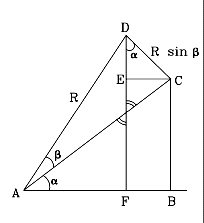# (M-11)  Deriving   sin(a+b),   cos(a+b)

Given the functions (sina, cosa, sinb and cos b), we seek formulas that express sin(a+b) and cos(a+b). The first of these formulas is used in deriving the L4 and L5 Lagrangian points, here.

### Please verify every calculation step before proceeding!As shown in the drawing, to derive the formula we combine two right-angled triangles

ABC which has an angle a
ABD which  "   "    "  b
The long side ("hypotenuse') of ACD is AD=R. Therefore

DC = R sin b
AC = R cos b
Similarly

BC = AC sin a = R cos b sin a
AB = AC cos a = R cos b cos a
The triangle ADF is right-angled and has the angle (a+b). Therefore

R sin (a+b) = DF
R cos (a+b) = AF

### Start by deriving the sine:

R sin (a+b) = DF  =  EF + DE  =  BC + DE
Note in the drawing the two head-to-head angles marked with double lines: like all such angles, they must be equal. Each of them is one of the two sharp ("acute") angles in its own right-angled triangle. Since the sharp angles in such a triangle add up to 90 degrees, the other two sharp angles must be equal. This justifies marking the angle near D as a, as drawn in the figure.

In the right-angled triangle CED

DE = DC cos a = R sin b cos a
EC = DC sin a = R sin b sin a
Earlier it was already shown that
BC = R cos b sin a
AB = R cos b cos a
Therefore

R sin (a+b)  =  BC+DE  =  R cos b sin a + R sin b cos a

Cancelling R and rearranging a to precede b

sin (a+b)  =  cos b sin a + sin b cos a

### Similarly, for the cosine

R cos (a+b) = AF = AB - FB = AB - EC =

= R cos b cos a - R sin b sin a

Cancelling R and rearranging

cos (a+b)  =  cos a cos b - R sin a sin b

Author and curator: David P. Stern, u5dps@lepvax.gsfc.nasa.gov
Last updated 25 February 1999